# Carnaval hacking 2017 : prog200-give_life_to_the_letters

Instructions on the attached file `README.md`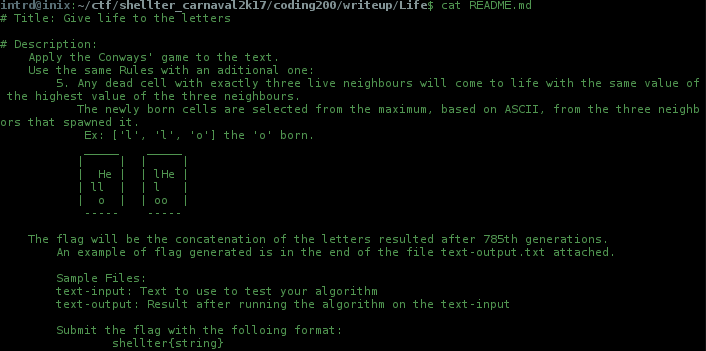## Solution

Well, this is a classic coding challenge, one of my favorite from this contest!

We've received a .7z file containing the `README.md` w/ the instructions above and some some other files..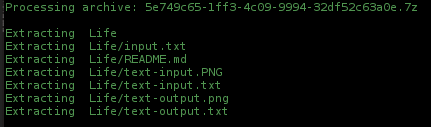`input.txt` A scrambled text file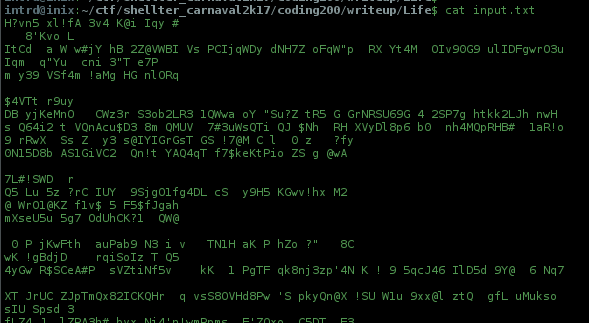`text-input.txt` Excerpt from Wikipedia - Conway's Game of Life (basic rules)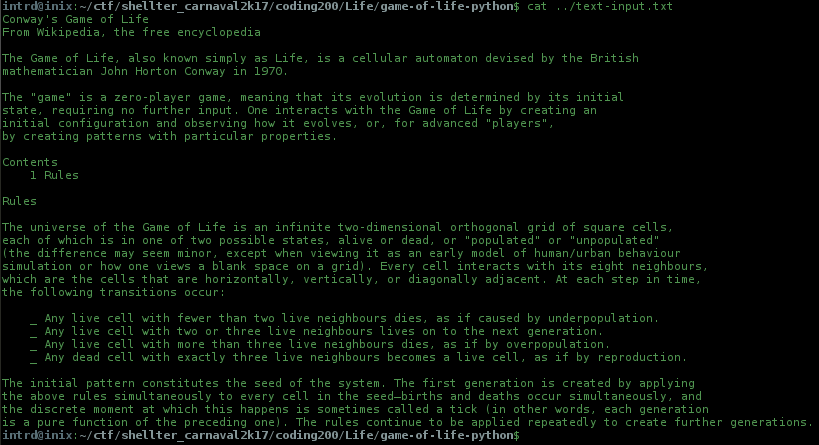`text-output.txt` Output of challenger GOL implementation, stopped @ 785 generation (using highest letters to represent cells following the 5th rule)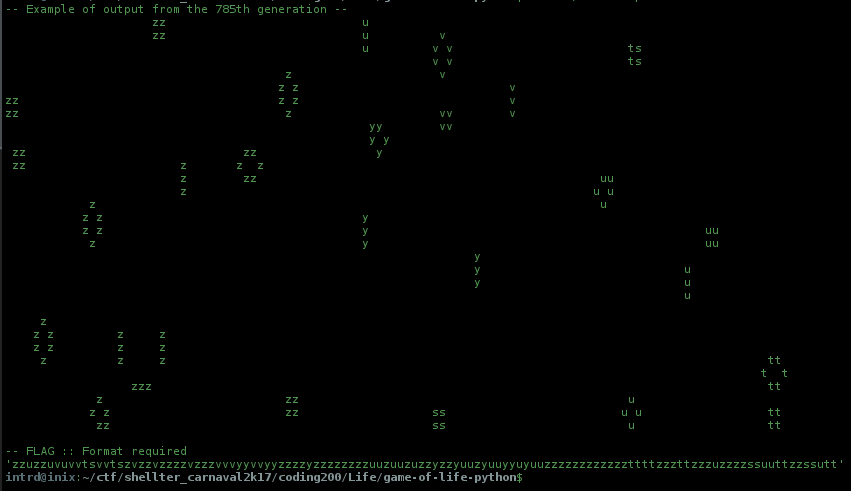Looking at the last line we can see the flag, it will be the concatenation of all remaining cells.

Obs: Comparing the content of `text-input.txt` and `text-output.txt` (excluding header/footer) we have the same number of lines and each line also has almost the same length of the original input.

Clearly what we need to do is..

TL;DR

1. Find a working GoL implementation (Preferably in python and that accepts a textfile as input)
2. Adapt this program to accept any ASCII character as cell
3. Add the 5th chall rule, and test w/ `Hello` glider sample provided in `README.md`
4. Convert `text-input.txt` to grid formatation, filling all null rows w/ empty spaces
5. Run the program, stop @ `785 generation`, extract and concatenate all remaining live cells, check if is the same of `text-output.txt`
6. Do the same w/ `input.txt`, format to `shellter{}`, post as the flag, profit!

Ok, it will be fun..

### Game of life python implementation

Searching at github, I found this py https://github.com/JulianLaval/game-of-life-python/blob/master/gameoflife.py, looks perfect, it accept text file as input following grid patterns like this..

• `0` = dead cells
• `1` = live cells
``````00000010000000000
00000001000000000
00000111000000000
00000000000000000
00000000000000000
``````

GoL's glider representation..

Running the original program..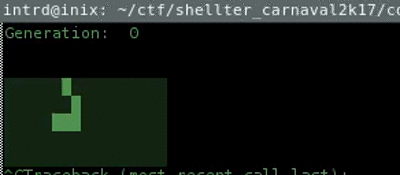This is perfect for our needs!

### Program adaptation

I started by modifying the program to accept any ASCII char instead only 0's and 1's.

`nextGen()`

``````def nextGen(inputArray):
newArray = deepcopy(inputArray)
for lineKey, line in enumerate(inputArray):
for charKey, char in enumerate(line):
if inputArray[lineKey][charKey] == "1" and neighbours(lineKey, charKey) < 2:
newArray[lineKey][charKey] = "0"
elif inputArray[lineKey][charKey] == "1" and neighbours(lineKey, charKey) > 3:
newArray[lineKey][charKey] = "0"
elif inputArray[lineKey][charKey] == "0" and neighbours(lineKey, charKey) == 3:
newArray[lineKey][charKey] = "1"
``````
``````def nextGen(inputArray):
newArray = deepcopy(inputArray)
for lineKey, line in enumerate(inputArray):
for charKey, char in enumerate(line):
if inputArray[lineKey][charKey] != " " and neighbours(lineKey, charKey) < 2:
newArray[lineKey][charKey] = " "
elif inputArray[lineKey][charKey] != " " and neighbours(lineKey, charKey) > 3:
newArray[lineKey][charKey] = " "
elif inputArray[lineKey][charKey] == " " and neighbours(lineKey, charKey) == 3:
newArray[lineKey][charKey] = "x"
``````

`neighbours()`

``````def neighbours(y, x):
counter = 0
try:
if x-1 >= 0 and y-1 >= 0 and inputArray[y-1][x-1] == "1":
counter += 1
except:
pass
try:
if x >= 0 and y-1 >= 0 and inputArray[y-1][x] == "1":
counter += 1
except:
pass
...
``````
``````def neighbours(y, x):
counter = 0
try:
if x-1 >= 0 and y-1 >= 0 and inputArray[y-1][x-1] != " ":
counter += 1
except:
pass
try:
if x >= 0 and y-1 >= 0 and inputArray[y-1][x] != " ":
counter += 1
except:
pass
...
``````

This way the program will understand that the `spaces = dead cells` and `any other character = live cells`

...added a `exit()` when it reachs `785` gen..

``````if gen == 785:
exit()
``````

To test, we need to format our original Glider to a space grid..

``````00000010000000000
00000001000000000
00000111000000000
00000000000000000
00000000000000000
original
``````
``````      X
X
XXX

formated
``````

running..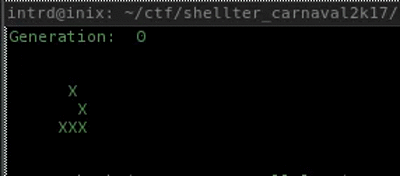### The 5th rule

With a working GoL implementation we need to add the 5th rule created by challengers..

Conway's Game of Life - Original rules

1. Any live cell with fewer than two live neighbours dies, as if caused by underpopulation.
2. Any live cell with two or three live neighbours lives on to the next generation.
3. Any live cell with more than three live neighbours dies, as if by overpopulation.
4. Any dead cell with exactly three live neighbours becomes a live cell, as if by reproduction.

... the new one

Any dead cell with exactly three live neighbours will come to life with the same value of the highest value of the three neighbours. The newly born cells are selected from the maximum, based on ASCII, from the three neighbors that spawned it.

So, at `nextGen()` we can see the 4 rules, I just changed the 4th rule to return `maxval` of neighbors arrays instead fixed "x" char.

``````def nextGen(inputArray):
newArray = deepcopy(inputArray)
for lineKey, line in enumerate(inputArray):
for charKey, char in enumerate(line):
if inputArray[lineKey][charKey] != " " and neighbours(lineKey, charKey) < 2:
newArray[lineKey][charKey] = " "
elif inputArray[lineKey][charKey] != " " and neighbours(lineKey, charKey) > 3:
newArray[lineKey][charKey] = " "
elif inputArray[lineKey][charKey] == " " and neighbours(lineKey, charKey) == 3:
#newArray[lineKey][charKey] = "x"
neis = get_neighbours(lineKey, charKey)
maxval=get_highest_value_from_array(neis)
newArray[lineKey][charKey] = maxval
``````

..new functions

``````## return `maxval` of neighbors arrays
def get_highest_value_from_array(arrayy):
return(max(arrayy))

## get neighbors values..
def get_neighbours(y, x):
counter = 0
neigs = []
try:
if x-1 >= 0 and y-1 >= 0 and inputArray[y-1][x-1] != " ":
neigs.append(inputArray[y-1][x-1])
#counter += 1
except:
pass
try:
if x >= 0 and y-1 >= 0 and inputArray[y-1][x] != " ":
neigs.append(inputArray[y-1][x])
#counter += 1
except:
pass
...
``````

Now go to `README.md` and convert the Hello Glider to our format..

`````` He
ll
o
``````

.. and run 1 generation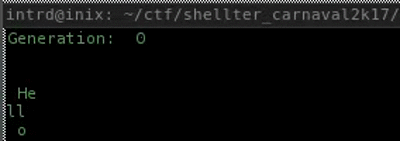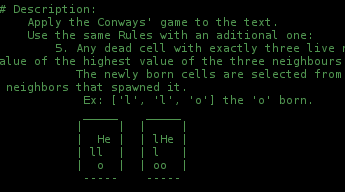Same, no?

Now let's simule w/ given sample `text-input.txt`

I created some rules to format the input file (by hand)

• The longest line dictates the grid length
• Fill all the blank spaces
• Add a line break after the last line

Yes, we can do this by code, but at the CTF we have no time!

`text-input.txt` formated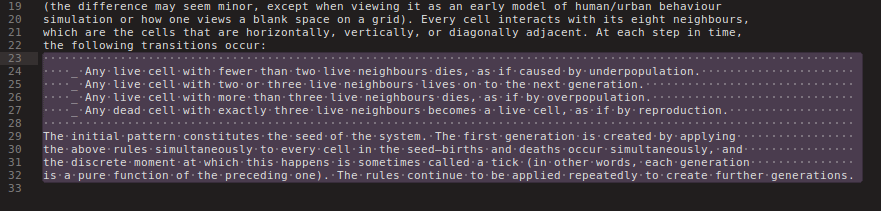### Running

Expected result..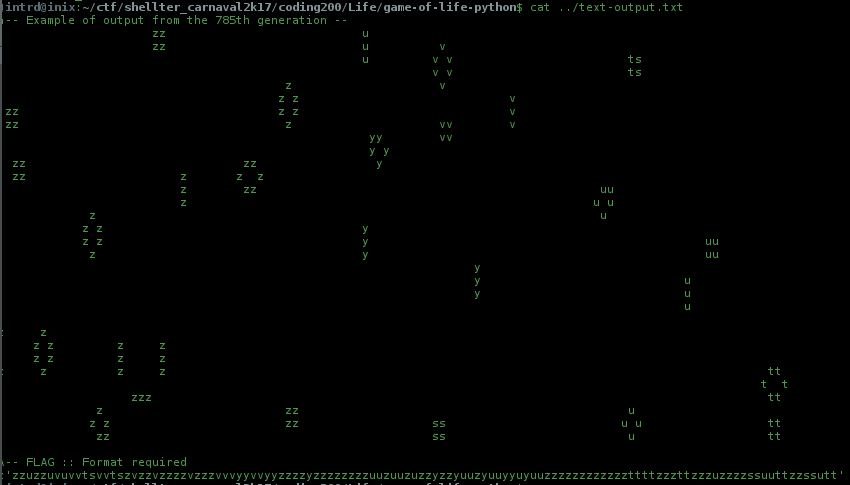My result at 485th gens..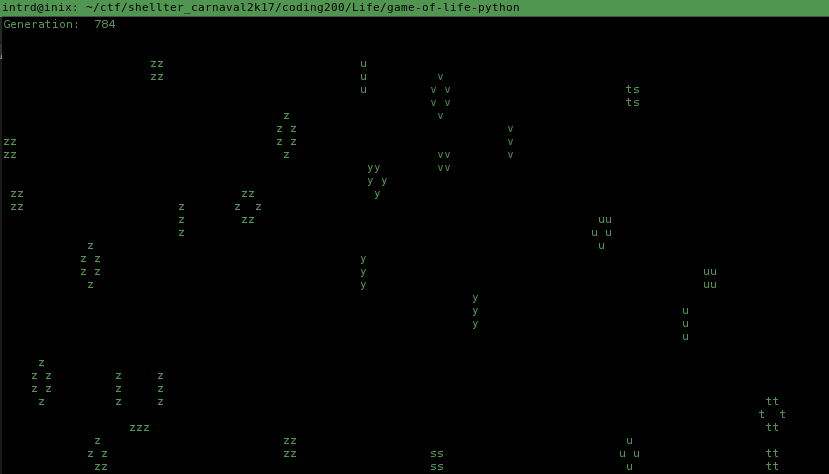Perfect!

And finally the same w/ given `input.txt`, the 485th gen is the flag!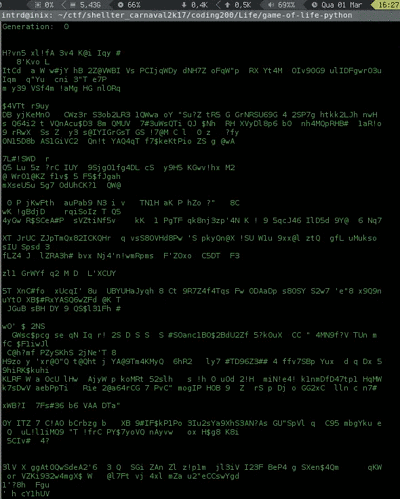Note: this gif is show only 457 generations, u need to run script by your own :)

`Flag: shellter{CENSORED}`

### Shellter Carnaval Hacking 2017 final score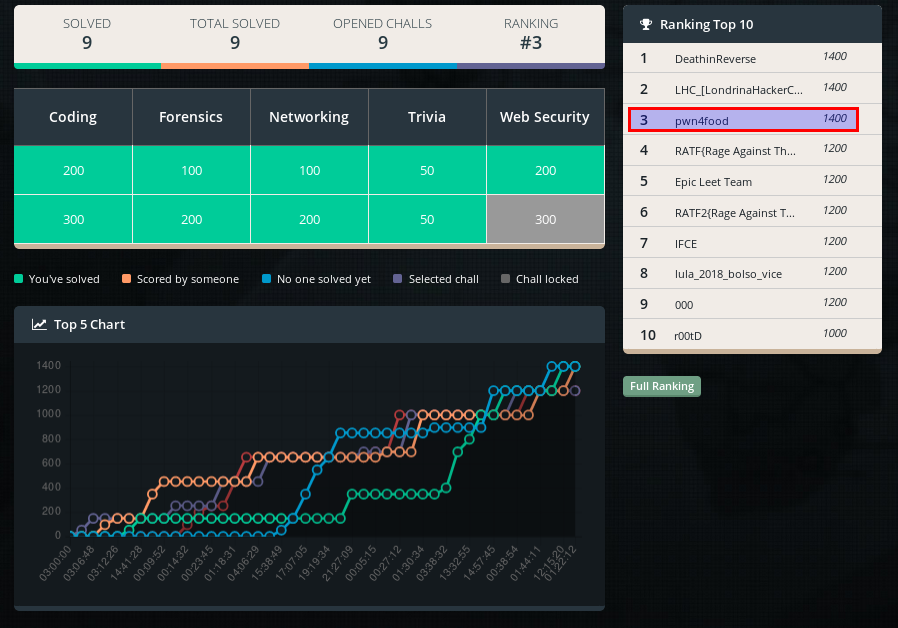## Helpful reading

comments powered by Disqus

Bitcoin Donate 3C1wt7a83sHf2AnutNZF22nXPZubGKdi2m